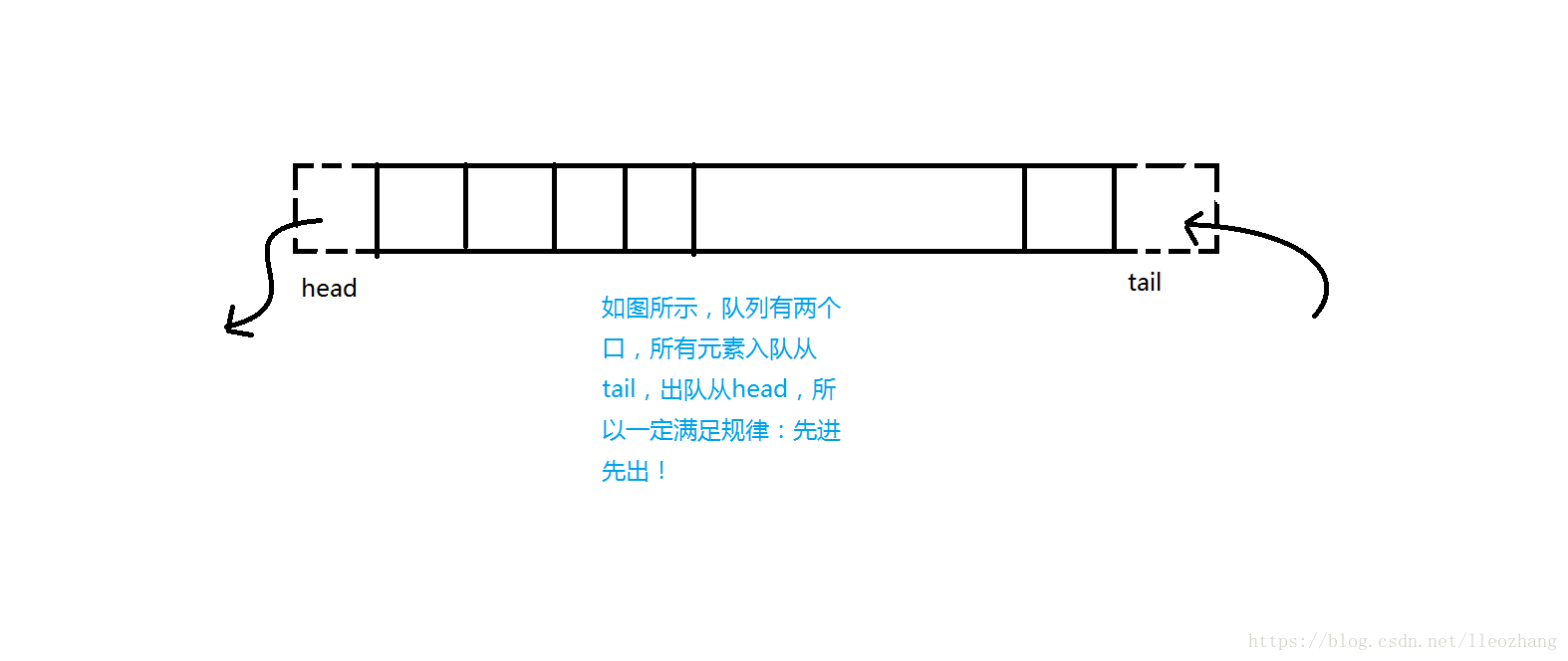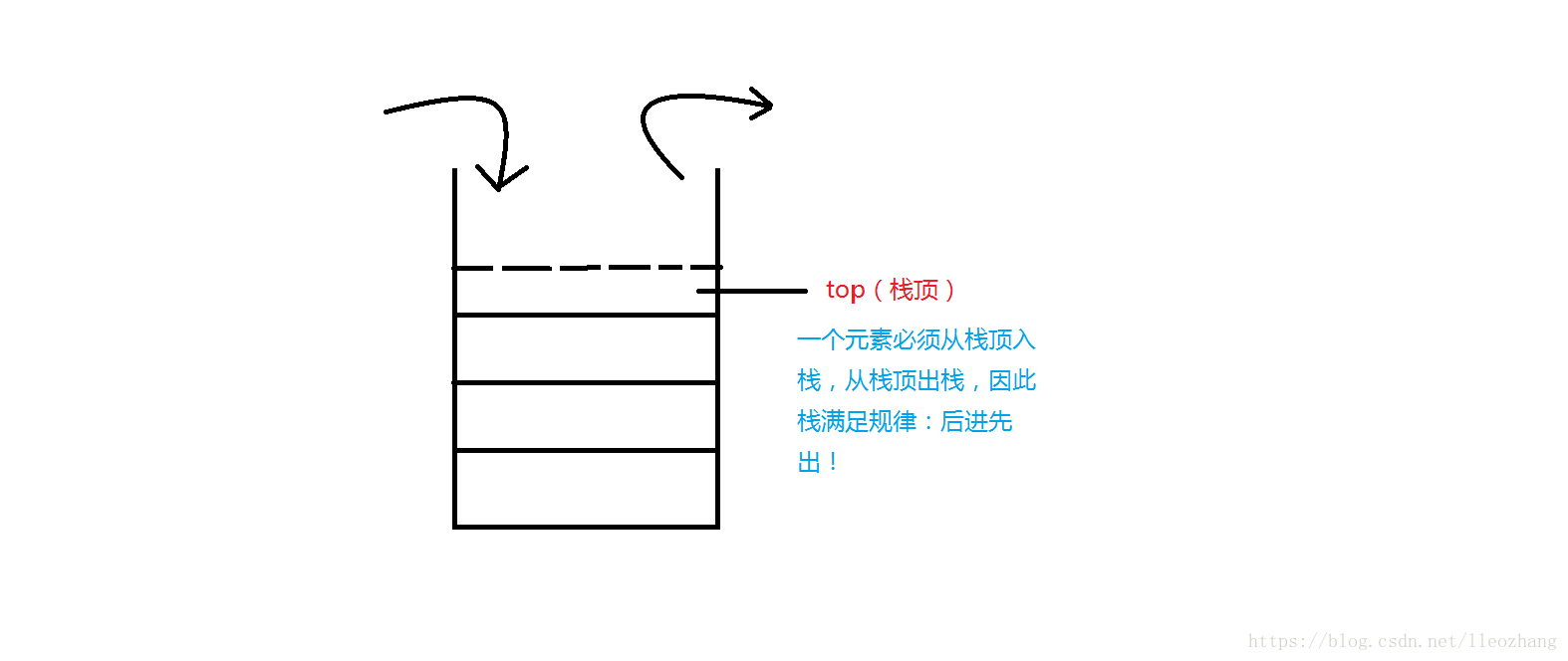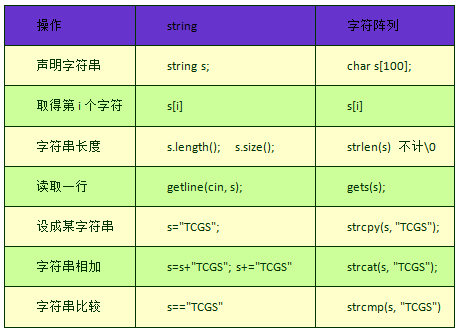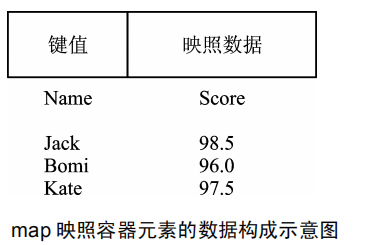2019/11/08 20:58

# 史上最全的C++ STL 容器大礼包

vector讲解传送门

## vector容器的概念

$vector$在英文中是矢量的意思。如果学过高中数学必修四的平面向量或者高中物理必修一的第一节课对其会有一个直观的认识。但是在$STL$中，$vector$和物理、几何等东西没有任何关系。

## vector容器的声明

$vector$容器存放在模板库：#include<vector>里，使用前需要先开这个库。

$vector$容器的声明遵循$C++STL$的一般声明原则：

#include<vector>
vector<int> vec;
vector<char> vec;
vector<pair<int,int> > vec;
vector<node> vec;
struct node{...};


## vector容器的使用方法

$vector$容器的使用方法大致如下表所示：

vec.begin(),vec.end() 返回vector的首、尾迭代器
vec.front(),vec.back() 返回vector的首、尾元素
vec.push_back() 从vector末尾加入一个元素
vec.size() 返回vector当前的长度（大小）
vec.pop_back() 从vector末尾删除一个元素
vec.empty() 返回vector是否为空，1为空、0不为空
vec.clear() 清空vector

# 浅谈C++ STL queue 容器

queue讲解传送门

## queue容器的概念

$queue$在英文中是队列的意思。队列是一种基本的数据结构。而$C++STL$中的队列就是把这种数据结构模板化了。我们可以在脑中想象买票时人们站的排队队列。我们发现，在一个队列中，只可以从队首离开，从队尾进来（没有插队，想啥呢）。即一个先进先出的数据结构。## queue容器的声明

$queue$容器存放在模板库：#include<queue>里，使用前需要先开这个库。

$queue$容器的声明遵循$C++STL$的一般声明原则：


#include<queue>

queue<int> q;

queue<char> q;

queue<pair<int,int> > q;

queue<node> q;

struct node{...};



## queue容器的使用方法

$queue$容器的使用方法大致如下表所示：

q.front(),q.back() 返回queue的首、尾元素
q.push() 从queue末尾加入一个元素
q.size() 返回queue当前的长度（大小）
q.pop() 从queue末尾删除一个元素
q.empty() 返回queue是否为空，1为空、0不为空

$queue$不支持随机访问，即不能像数组一样地任意取值。并且，$queue$并不支持全部的$vector$的内置函数。比如$queue$不可以用$clear()$函数清空，清空$queue$必须一个一个弹出。同样，$queue$也并不支持遍历，无论是数组型遍历还是迭代器型遍历统统不支持，所以没有$begin(),end();$函数，使用的时候一定要清楚异同！

# 浅谈C++ STL stack 容器

stack讲解传送门

## stack容器的概念

$stack$在英文中是栈的意思。栈是一种基本的数据结构。而$C++STL$中的栈就是把这种数据结构模板化了。 栈的示意图如下：这是一个先进后出的数据结构。这非常重要！！事实上，$stack$容器并不是一种标准的数据结构，它其实是一个容器适配器，里面还可以存其他的$STL$容器。但那种使用方法过于高深而且不是很常用，所以在此不与介绍。请有兴趣的读者自行查询资料。

## stack容器的声明

$stack$容器存放在模板库：#include<stack>里，使用前需要先开这个库。 $stack$容器的声明遵循$C++STL$的一般声明原则： 容器类型<变量类型> 名称 例：

#include<stack>
stack<int> st;
stack<char> st;
stack<pair<int,int> > st;
stack<node> st;
struct node{...};


## stack容器的使用方法

$stack$容器的使用方法大致如下表所示： | 用法 | 作用 | | ------------ | --------------------------------- | | st.top() | 返回stack的栈顶元素 | | st.push() | 从stack栈顶加入一个元素 | | st.size() | 返回stack当前的长度（大小） | | st.pop() | 从stack栈顶弹出一个元素 | | st.empty() | 返回stack是否为空，1为空、0不为空 |

# 浅谈C++ STL string容器

## string容器的使用方法及与传统字符读入的对比# 详解C++ STL priority_queue 容器

## priority_queue容器的概念

$priority_queue$在英文中是优先队列的意思。## priority_queue容器的声明

$priority_queue$容器存放在模板库：#include<queue>里，使用前需要先开这个库。

#### 大根堆声明方式：

#include<queue>
priority_queue<int> q;
priority_queue<string> q;
priority_queue<pair<int,int> > q;


$C++$中的$int,string$等类型可以直接比较大小，所以不用我们多操心，优先队列自然会帮我们实现。但是如果是我们自己定义的结构体，就需要进行重载运算符了。关于重载运算符的讲解，请参考这篇博客：

#### 小根堆声明方式

priority_queue<int,vector<int>,greater<int> >q;


## priority_queue容器的使用方法

$priority_queue$容器的使用方法大致如下表所示：

q.top() 返回priority_queue的首元素
q.push() 向priority_queue中加入一个元素
q.size() 返回priority_queue当前的长度（大小）
q.pop() 从priority_queue末尾删除一个元素
q.empty() 返回priority_queue是否为空，1为空、0不为空

# 浅谈C++ STL deque 容器

deque讲解传送门

## deque容器的概念

$deque$的意义是：双端队列。队列是我们常用而且必须需要掌握的数据结构。$C++STL$中的确有模拟队列的模板：#include<queue>中的$queue$和$priority_queue$。队列的性质是先进先出，即从队尾入队，从队首出队。而$deque$的特点则是双端进出，即处于双端队列中的元素既可以从队首进/出队，也可以从队尾进/出队。

$deque$模板存储在$C++STL$的#include<deque>中。

## deque容器的使用方法

q.begin(),q.end() 返回deque的首、尾迭代器
q.front(),q.back() 返回deque的首、尾元素
q.push_back() 从队尾入队一个元素
q.push_front() 从队头入队一个元素
q.pop_back() 从队尾出队一个元素
q.pop_front() 从队头出队一个元素
q.clear() 清空队列

# 详解C++ STL set 容器

set讲解传送门

## set容器的概念和性质

$set$在英文中的意义是：集合。$set$容器也的确“人如其名”，实现了这个集合的功用。

## set容器的声明

$set$容器的声明和大部分$C++STL$容器一样，都是：容器名<变量类型> 名称的结构。前提需要开#include<set>库。如：

#include<set>
set<int> s;
set<char> s;
set<pair<int,int> > s;
set<node> s;
struct node{...};


## set容器的使用

s.empty();


$empty()$函数返回当前集合是否为空，是返回1，否则返回0.

s.size();


$size()$函数返回当前集合的元素个数。

s.clear();


$clear()$函数清空当前集合。

s.begin(),s.end();


$begin()$函数和$end()$函数返回集合的首尾迭代器。注意是迭代器。我们可以把迭代器理解为数组的下标。但其实迭代器是一种指针。这里需要注意的是，由于计算机区间**“前闭后开”**的结构，$begin()$函数返回的指针指向的的确是集合的第一个元素。但$end()$返回的指针却指向了集合最后一个元素后面一个元素。

s.insert(k);


$insert(k)$函数表示向集合中加入元素$k$。

s.erase(k);


$erase(k)$函数表示删除集合中元素$k$。这也反映了$set$容器的强大之处，指哪打哪，说删谁就删谁，完全省略了遍历、查找、复制、还原等繁琐操作。更不用像链表那种数据结构那么毒瘤。直接一个函数，用$O(logn)$的复杂度解决问题。

s.find(k);


$find(k)$函数返回集合中指向元素$k$的迭代器。如果不存在这个元素，就返回$s.end()$，这个性质可以用来判断集合中有没有这个元素。

## 其他好用的函数

s.lower_bound(),s.upper_bound();


s.equal_range();


# 详解C++ STL multiset 容器

multiset讲解传送门

## multiset容器的概念和性质

$set$在英文中的意义是：集合。而$multi-$前缀则表示：多重的。所以$multiset$容器就叫做：有序多重集合。

$multiset$的很多性质和使用方式和$set$容器差不了多少。而$multiset$容器在概念上与$set$容器不同的地方就是：$set$的元素互不相同，而$multiset$的元素可以允许相同。

C++STL set容器详解

## 与set容器不太一样的地方：

s.erase(k);


$erase(k)$函数在$set$容器中表示删除集合中元素$k$。但在$multiset$容器中表示删除所有等于$k$的元素。

if((it=s.find(a))!=s.end())
s.erase(it);


$if$中的条件语句表示定义了一个指向一个$a$元素迭代器，如果这个迭代器不等于$s.end()$，就说明这个元素的确存在，就可以直接删除这个迭代器指向的元素了。

s.count(k);


$count(k)$函数返回集合中元素$k$的个数。$set$容器中并不存在这种操作。这是$multiset$独有的。

# C++ STL bitset 容器详解

bitset讲解传送门

## $bitset$容器概论

$bitset$容器其实就是个$01$串。可以被看作是一个$bool$数组。它比$bool$数组更优秀的优点是：**节约空间，节约时间，支持基本的位运算。**在$bitset$容器中，$8$位占一个字节，相比于$bool$数组$4$位一个字节的空间利用率要高很多。同时，$n$位的$bitset$在执行一次位运算的复杂度可以被看作是$n/32$，这都是$bool$数组所没有的优秀性质。

$bitset$容器包含在$C++$自带的$bitset$库中。

#include<bitset>


## $bitset$容器的声明

bitset<100000> s;


## 对$bitset$容器的一些操作

#### 1、常用的操作函数

• $count()$函数

$count$，数数的意思。它的作用是数出$1$的个数。即$s.count()$返回$s$中有多少个$1$.

s.count();

• $any()/none()$函数

$any$，任何的意思。$none$，啥也没有的意思。这两个函数是在检查$bitset$容器中全$0$的情况。

s.any();
s.none();

• $set()$函数

$set()$函数的作用是把$bitset$全部置为$1$.

s.set();
s.set(u,v);

• $reset()$函数

s.reset();
s.reset(k);

• $flip()$函数

$flip()$函数与前两个函数不同，它的作用是将整个$bitset$容器按位取反。同上，其传进的参数表示把其中一位取反。

s.flip();
s.flip(k);


#### 2、位运算操作在$bitset$中的实现

$bitset$的作用就是帮助我们方便地实现位运算的相关操作。它当然支持位运算的一些操作内容。我们在编写程序的时候对数进行的二进制运算均可以用在$bitset$函数上。

~：按位取反

&：按位与

|：按位或

^：按位异或

<< >>：左/右移

==/！=：比较两个$bitset$是否相等。

s=1;
s=0;


## $bitset$容器的实际应用

$bitset$可以高效率地对$01$串，$01$矩阵等等只含$0/1$的题目进行处理。其中支持的许多操作对我们处理数据非常有帮助。如果碰到一道$0/1$题，使用$bitset$或许是不错的选择。

# 详解C++ STL map 容器

map讲解传送门

## map容器的概念

$map$的英语释义是“地图”，但$map$容器可和地图没什么关系。$map$是**“映射容器”**，其存储的两个变量构成了一个键值到元素的映射关系。$map$容器的内部实现是一棵红黑树（平衡树的一种），因为比较复杂而且与理解并无多大关系，所以不予介绍，有兴趣的读者可以自己查阅相关的资料。

## map容器的声明

$map$容器存在于$STL$模板库#include<map>中。使用的时候需要先开这个库。

#include<map>
map<int,char> mp;


## map容器的用法

bitset容器详解

set容器详解

#### 插入操作

$map$容器的插入操作大约有两种方法，第一种是类似于数组类型，可以把键值作为数组下标对$map$进行直接赋值：

mp='a';


mp.insert(map<int,char>::value_type(5,'d'));


#### 删除操作

mp.erase('b');


#### 查找操作

mp.find(1);


## map和pair的关系

@JZYShruraK大佬

0
0 收藏

### 作者的其它热门文章0 评论
0 收藏
0